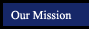LAUSD Superintendent John Deasy has forced me to remove all my advertising to keep my job as a teacher. Soon I will have to close the website without the ad revenue. Please sign my petition to the Superintendent so I may put ads back on my website and keep it open. You can also email him at Superintendent@LAUSD.net Thanks, Lisa Gehman Thorne

CAHSEE Prep - 2008 Released Test Questions: Number Sense
 Navigate by Topic Reference Textbooks Topic 1: Number Sense Topic 2: Statistics, Data Analysis and Probability Topic 3: Algebra and FunctionsMathematics Concepts and Skills Course 1Prentice/Hall Pre-Algebra Topic 4: Measurement and Geometry Topic 5: Mathematical Reasoning Topic 6: Algebra 1Mathematics Concepts and Skills Course 2Algebra 1 Concepts and Skills
Number Sense
Read, write, and compare rational numbers in scientific notation (positive and negative powers of 10) with approximate numbers using scientific notation. 7NS1.1
Released Test Questions (CAHSEE Revolution Question Numbers) 1(1) 2 3
 Practice Games Scientific Notation Game - Changing Numbers to Scientific Notation Convert scientific notation to decimal numbers - AAAmath Convert scientific notation to large numbers - 321Know.com
Add, subtract, multiply, and divide rational numbers (integers, fractions, and terminating decimals) and take positive rational numbers to whole-number powers. 7NS1.2
Released Test Questions (CAHSEE Revolution Question Numbers)       4(2) 5 6 7 8
 Integers Practice, Examples and Games Fractions Practice, Examples and Games Decimals Practice, Examples and Games Exponents Practice, Examples and Games

Convert fractions to decimals and percents and use these representations in estimations, computations, and applications. 7NS1.3
Released Test Questions (CAHSEE Revolution Question Numbers)                 9(3) 10
11
 Practice Games Fraction to Decimal - 321Know.com Decimal to Percent - 321Know.com Matching Common Decimals and Fractions - 321Know.com Percent to Fraction - 321Know.com Percent to Decimal - 321Know.com Fraction to Percent - 321Know.com
Calculate the percentage of increases and decreases of a quantity. 7NS1.6
Released Test Questions (CAHSEE Revolution Question Numbers)
12(4) 13 14
 Practice Games coming soon... try again later!
Solve problems that involve discounts, markups, commissions, and profit, and compute simple and compound interest. 7NS1.7
Released Test Questions (CAHSEE Revolution Question Numbers)         15(15) 16 17 18 19
 Practice Games Discount - 321Know.com Markup - 321Know.com Total Price with Tax - 321Know.com Simple Interest - 321Know.com
Understand negative whole-number exponents. Multiply and divide expressions involving exponents with a common base. 7NS2.1
Released Test Questions (CAHSEE Revolution Question Numbers)                   20(6)
21 22
 Practice Games
Add and subtract fractions by using factoring to find common denominators. 7NS2.2
Released Test Questions  (CAHSEE Revolution Question Numbers)     23 24(7) 25
Fractions Practice, Examples and Games
Multiply, divide, and simplify rational numbers by using exponent rules. 7NS2.3
Released Test Questions  (CAHSEE Revolution Question Numbers)           26(8) 27 28 29
 Practice Games Using Exponent Rules Practice Game
Use the inverse relationship between raising to a power and extracting the root of a perfect square integer; for an integer that is not square, determine without a calculator the two integers between which its square root lies and explain why. 7NS2.4
Released Test Questions  (CAHSEE Revolution Question Numbers)                   30(9)
31 32
 Practice Games Matching Perfect Square Roots - Quia.com Find Square Root Practice - SoftSchools.com Square Root Flashcards - Aplusmath.com
Understand the meaning of the absolute value of a number; interpret the absolute value as the distance of the number from zero on a number line; and determine the absolute value of real numbers. 7NS2.5
Released Test Questions  (CAHSEE Revolution Question Numbers)     33(10) 34 35
 Practice Games Absolute Value - AAAMath.comMath Glossary
(more sophisticated than it looks!)